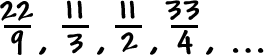Now that we know how these geometric guys work, we won't have to do the table thing anymore!

What are all the pieces?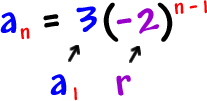Here's the general formula:

 Given a geometric sequence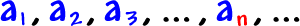with a ratio of r, the nth term is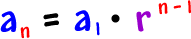Let's try it!

What's the 23th term of this sequence?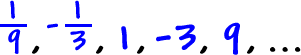Find the ratio: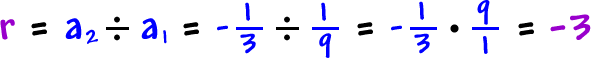So...By the way, these (  ) are really important if your ratio is negative!       So, be careful!

TRY IT:

Find the formula for the nth term, then use it to find the 11th term of this sequence